• 2022-01-04 21:59:12

## Log

2022.01.04今天先开个头，明天继续
2022.01.05继续弄感觉好理解，但是东西有点多
2022.01.06白天要搞别的事，晚上来学
2022.01.07收个尾

# 一、基本操作（basic operations）

## 1.基本运算

• 注释用百分号%
• help functionName：显示指定函数的帮助函数。
• exit or quit ： 退出 Octave。

### ①加减乘除幂

• 5 + 6 ⇒ 11
• 3 - 2 ⇒ 1
• 5 * 8 ⇒ 40
• 1 / 2 ⇒ 0.50000
• 2 ^ 6 ⇒ 64

### ②逻辑运算

• 相等：1 == 2 ⇒ 0
1 等于 2 判断为假，false 用 0 表示
• 不等：1 ~= 2 ⇒ 1
1 不等于 2 判断为真，true 用 1 表示
• 逻辑与：1 && 0 ⇒ 0
• 逻辑或：1 || 0 ⇒ 1
• 异或运算：XOR(1,0) ⇒ 1

### ③更改提示符

• 一般Octave提示符显示版本以及其它信息，可以通过PS1(">>")语句将其改为>>

## 2.变量操作

### ①变量赋值

• a = 3 变量 a 赋值为 3 ，屏幕显示。
a = 3; 变量 a 赋值为 3 ，末尾添加分号（semicolon），屏幕不显示。
• b = 'hi'字符串赋值
• c = (3>=1) 表达式赋值

### ②变量查询/输出信息

• 可以通过输入变量名直接查询其存储的信息：
a = pi;aa = 3.1416
• 通过disp函数输出相应数值：
a = pi;disp(a)3.1416
• 通过更加复杂的方式输出字符串：
disp(sprintf('2 decimals: %0.2f', a))decimals: 3.14
disp(sprintf('6 decimals: %0.6f', a))decimals: 3.141593
• 字符串显示默认的位数：
format longaa = 3.14159265358979
• 打印少量的小数点后位数：
format shortaa = 3.1416

## 3.向量和矩阵

### ①建立矩阵

• 创建矩阵：A = [1 2; 3 4; 5 6]
等价方式：A = [1 2;3 4;5 6]
（分号让矩阵换到下一行）
• 行向量：V = [1 2 3]
• 列向量：V = [1; 2; 3]

### ②生成矩阵的快捷方法

• V = 1:0.1:2
说明：行向量 V 从 1 开始，0.1 为步长，到 2 停止，即建立一个 1×11 维的矩阵
• V = 1:6
说明：行向量V = [1 2 3 4 5 6]
• zeros(1,3)
说明：建立一个 1×3 的矩阵，每个元素都为 0
• ones(2,3)
说明：建立一个 2×3 的矩阵，每个元素都为 1
• C = 2 * ones(2,3)
说明：建立一个 2×3 的矩阵 C，每个元素都为 2
• rand(m,n)
说明：随机生成一个 m×n 维的矩阵，每个元素介于 0 和 1 之间
• randn(m,n)
说明：随机生成一个 m×n 维的矩阵，且所有元素服从高斯分布/正态分布（Gaussian/Normal distribution），均值（mean）为0标准差（variance）或者方差（deviation）为1

### ③绘制矩阵直方图

• w = -6 + sqrt(10) * (randn(1,10000))
• hist(w)
绘制直方图（histogram）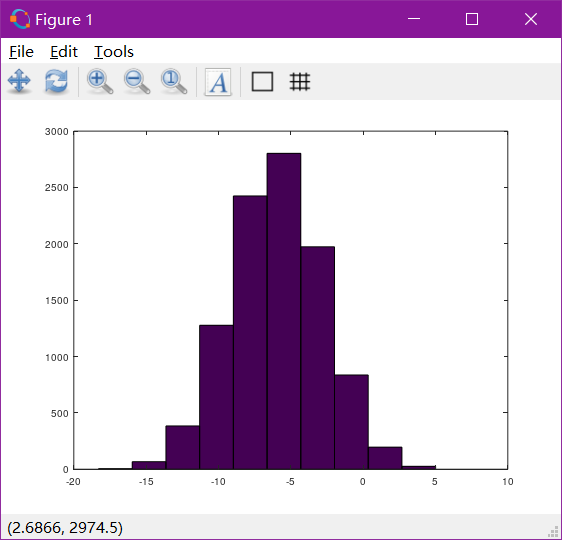• hist(w,50)
更多竖条（buckets） 的直方图：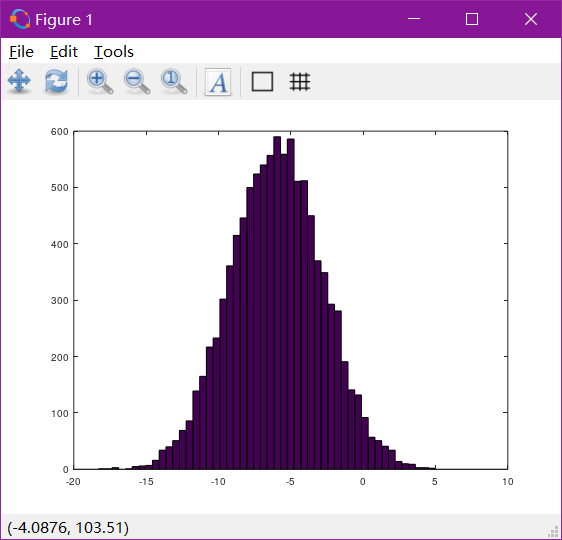### ④生成矩阵的特殊方法

• eye（n）：生成 n 阶单位矩阵

# 二、移动数据（move data around）

## 1.计算矩阵大小

• A = [1 2; 3 4; 5 6]
• size(A)ans = 3 2
表示 A 的大小为 3×2，同时，该结果也可以看作是一个新的 1×2 维的矩阵，即：
size(size(A))ans = 1 2
• size(A,1)：返回 A 的第一维度大小，即行数
• size(A,2)：返回 A 的第二维度大小，即列数
• length(A)：返回 A 的最大维度大小

## 2.在文件系统中加载和查找数据

### ①数据加载/保存

• pwd：显示当前所在路径
• cd：更改路径
• ls：列出当前路径下的文件
• load fileName or load('fileName') ：将指定文件加载到 Octave 中（文件中的所有内容被看做是一个变量）
• who ：显示当前 Octave 中储存的所有变量
• whos ：显示当前 Octave 中储存的所有变量的详细信息（包括变量名、维度大小、内存大小、变量类型）
• clear v ： 删除变量 v
• clear ： 删除内存中所有内容
• A = B(1:10) ： 将 B 的前 10 个元素赋给 A（超出范围会有报错）
• save hello.mat v ： 将变量 v 保存到文件 hello.mat 中（文件不存在会自动创建，重复写入不同信息会覆盖原有内容，这里就可以发现和加载文件的命令是存在着一定关联的）
• save hello.txt v -ascii ： 数据储存成 ASCII 编码的文档（即文本文档），不加最后一个字段默认是以二进制存储，如果数据很大还会对其进行压缩（咱觉得是用一种编码压缩的方式，加载的时候还会进行解码）

### ②数据查找/矩阵赋值/矩阵合成

A 是一个 3×2 维的矩阵

• A(3,2) ：查找矩阵 A 中第三行第二列的元素（和从0开始的数组下标索引不同）
• A(2,:) ：查找矩阵 A 中第二行的所有元素，此处 : 代表该行的所有元素（也可以用来代表一列，如 A(:,2)
• A([1 3],:) ：第一索引为 1 ，第二索引为 3 ，即查找 A 中第 1 行和第 3 行的所有元素
• A(:,2) = [10; 11; 12] ： 对 A 的第二列数据进行重新赋值
• A = [A, [100; 101; 102]] ： 在 A 后面添加一列数据（感觉和字符串很像：str = str + ‘s’）。当然，如果数据与维数不匹配是会报错的。
• A = [A; [100; 101]] ： 以此类推，把 , 换成 ; 就是添加新行。
• A(:) ： 把 A 放入一个单独的向量中
• C = [A B] / C = [A; B] ：合成 A B （左右/上下）

# 三、计算数据（do computational operations on data）

## 1.矩阵基本运算

• A * B ： 矩阵 A 与矩阵 B 相乘
• A .* B ： 矩阵 A 中的每一个元素与矩阵 B 中对应的元素相乘（一一对应）， . 点号（period） 表示对元素（element-wise） 的运算
• -A <==> -1 * A
• A .^ 2 ：A 中每个元素平方（squaring） （“ ^ ”，caret，脱字符号 （中文尚无通用名称）
• 1 ./ A ：A 中每个元素取倒数（inverse）
• log(A) ：对 A 中每个元素进行对数运算（logarithm）
• exp(A) ：以 e 为底，A 中元素为指数的幂运算（exponentiation）
• abs(A) ： 求 A 中所有元素的绝对值（absolute value）
• v + ones(length(v),1) <==> v + 1 ： v 中每个元素加 1（矩阵向量皆可）
• A + ones(size(A))<= =>A + ones(length(v),1) <= => A + ones(length(v),2) <==> A + 1 ： 同上（A 为 3×2 矩阵，A + ones(length(v),3)就不行，不是很理解A + ones(length(v),1)加一个 3×1 的矩阵可以把所有 3×2 的元素都加上）

## 2.矩阵其他运算

• A' ： 求 A 的转置（transpose），（A’——A prime）（'，撇号，apostrophe symbol，左引号，left quote）
• val = max(A) ： 返回 A 中最大元素的值
• [val, ind] = max(A) ： val 为中最大元素的值，ind 为最大值的索引（试了一下， A 为向量时还比较好理解，A 为 3×2 的矩阵时 val 是一个 1×2 维的向量，不是很理解）
• A < 3 ： 让 A 的每个元素进行比较，返回一个相同维度的矩阵，对应位置用 0 和 1 表示真假。
• find(a < 3) : 返回满足条件元素的下标索引
（嘿嘿，Markdown中<3⇒ ❤️ 。有意思）
以下 A 为 3 阶矩阵
• magic(n) ： 返回名为幻方（magic square） 的 n 阶矩阵，任意行、列、对角线中的元素相加值相同（每次 Octave 给出的 n 阶幻方都是固定的，不知道阶数确定时幻方是不是唯一的）
• [r, c] = find(A >= 7) ： r 为行地址索引，c 为列地址索引
• sum(a) ： 对 a 中所有元素求和
• sum(A, 1) ： 对 A 的每一列求和
• sum(A, 2) ： 对 A 的每一行求和
• prod(a) ： 返回所有元素的 乘积（product）
• floor(a) ： 所有元素向下取整（舍去小数部分）
• ceil(a) ： 向上取整（向上最接近的整数）
• max(rand(3),rand(3)) ： 返回取二者中元素较大者构成的一个新矩阵
• max(A, [], 1) ：得到每一列的最大值，其中 1 代表从第 1 维度取值
• max(A, [], 2) ：得到每一行的最大值，其中 2 代表从第 2 维度取值
• max(A) ：默认求每一列的最大值
• max(max(A)) ：求 A 中元素的最大值
• max(A(:)) ：同上，A(:) 表示 A 以一个向量的形式调用
• sum(sum(A. *eye(9))) ： 对矩阵的对角线进行求和运算
• flipud(A) ： 使矩阵垂直翻转（flip up down）
• sum(sum(A. *flipud(eye(9)))) ： 对矩阵的对角线进行求和运算

# 四、数据绘制（plotting data）

下面将要介绍一些 Octave 工具来绘图并可视化数据（plotting and visualizing data）

• 先初始化一点数据：

• t = [0: 0.01: 0.98];
• y1 = sin(2*pi*4*t);
• y2 = cos(2*pi*4*t);
• 绘制正弦函数（sine function）plot(t, y1);

• 绘制余弦函数（cosine function）plot(t, y2);

• 使两条图像重合的方式如下：

• plot(t, y1);
• hold on; ： 保留原有图像，在其基础上继续画（一次就足够，后面再加函数原图像仍然保留）
• plot(t, y2, 'r');'r' 使该曲线为红色（red），加以区分
• 继续对当前图像进行处理：

• xlabel('time') ： 添加 x 轴标签（label）
• ylabel('value') ： 添加 y 轴标签（label）
• legend('sin', 'cos') ： 标记（label）当前的两个函数，将图例（legend） 放在右上角（upper right）
• title('my plot') ： 在图像（figure）的顶部添加标题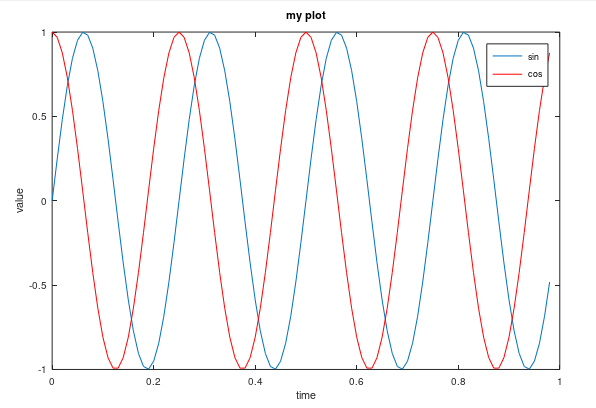• 最后还可以保存当前图像：print -dpng 'myPlot.png'

• 可以通过 help plot 查看将图像保存为其他格式的方法

• close ： 关闭图像

• 为图像标号（specify a figure numbers）:

• figure(1); plot(t, y1);
• figure(2); plot(t, y2);
• subplot(1, 2, 1); ： 将图像分为一个1*2的格子（参数1和参数2），使用第一个格子（参数3）

• axis([0.5 1 -1 1]) ： 设置 x 轴和 y 轴的范围

• clf; ： 清除一幅图像

• imagesc(A) ：绘制矩阵，不同颜色代表不同的值

• imagesc(A), colorbar, colormap gray; ： 生成一个彩色图像、一个灰度分布图，并在图像右侧加入一个颜色条，展示不同深度的颜色对应的数值。可以通过 , 逗号将多条语句写入同一行中。

# 五、控制语句（control statements）

## 1. for 语句

• v = zeros(10, 1)
• for i=1:10, ：从 1 遍历到 10
v(i) = 2^i;
end;
• 也可以这样写从 1 到 10 的循环：
indices = 1:10;
for i=incides,
disp(i);
end;
• breakcontinue 在 Octave 中也可以使用

## 2. while 语句

• i = 1;
while i <= 5,
v(i) = 100;
i = i + 1;
end;
• 用上了 if 和 break：
i = 1;
while true,
v(i) = 999;
i = i + 1;
if i == 6,
break;
end;
end;

## 3. if-else 语句

• if v(1)==1,
disp('The value is one');
elseif v(1)==2,
disp('The value is two');
else,
disp('The value is not one or two');
end;

## 4. 定义并使用函数

### ①基本

• 预先建立后缀为 .m 的文件，并以函数名作为文件名： functionName.m，例如 squareThisNumber(x) 。编辑其内容最好用文本编辑器进行（因为默认的记事本打开可能会把间距弄得很乱）
• 函数内容的说明：
function y = squareThisNumber(x) ： 返回一个值存到 y 里，且函数有一个自变量 x
y = x^2 ：定义函数的主体
• 只要文件在 Octave 当前的路径下，就可以直接通过函数名调用函数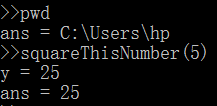• 除此之外， Octave 还允许定义的函数有多个返回值，如：
function [y1, y2] = squareAndCubeThisNumber(x)
y1 = x^2;
y2 = x^3;
• 再来一个稍微复杂一点的例子，如下图给出训练集求代价函数：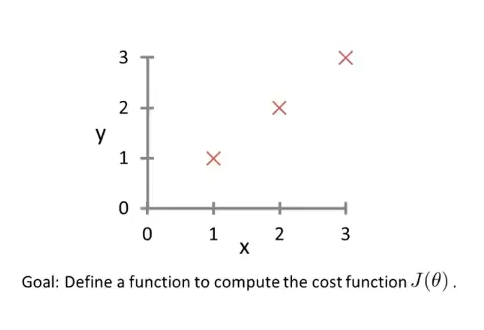• 首先在 Octave 中初始化数据：
X = [1 1; 1 2; 1 3]
y = [1; 2; 3]
theta = [0; 1]
• 定义函数 costFunctionJ.m
function J = costFunctionJ(X, y, theta)

% X is the "design matrix" containing our training examples.
% y is the class labels

m = size(X, 1);					% number of training examples
predictions = X*theta;			% predictions of hypothesis on all m examples
sqrErrors = (predictions-y).^2; % squared errors

J = 1/(2*m) * sum(sqrErrors);

• 调用函数：
j = costFunctionJ(X, y, theta)
• 得到的结果为 j = 0 ，表示完美拟合
• 如果 theta = [0; 0] ，得到结果为 j = 2.3333 ，说明并不能很好的拟合，该结果实际上就是每个样本的平方差之和再除以 2m ，即 (1^2 + 2^2 + 3^2) / (2*3)

### ②进阶

• addpath('C:\path\...') ： 将指定路径添加到 Octave 的搜索路径，当切换到其他路径时仍然可以调用添加的路径下的函数（只在一次中有效，即退出 Octave 重新进入时需要重新添加路径）

# 六、向量化（vectorization）

## 1.简述

• Octave 、 MATALAB、 Python NumPy、Java、 C、 C++这些语言都具有内置的或者易获取的各种线性代数库（linear algebra libraries） ， 都已经实现了高度优化，如果能够利用好他们（或者说数值（numerical） 线性代数库），并联合调用他们（mix the routine calls to them），即用好函数库不用自己手写：
1. 用内置运行速度会更快，并且可以有效的利用计算机里可能有的一些并行硬件系统（parallel hardware）
2. 使用更少的代码实现需要的功能，因此实现的方式更简单，更不容易出错 （be bug free）
• 如果使用了合适的向量化方法（vectorized implementations） ， 代码会简单有效得多，下面举个例子：

## 2.实例

### ①简单例子——计算线性回归假设函数

• 计算线性回归假设函数：
h θ ( x ) = ∑ j = 0 n θ j x j = θ T x θ = [ θ 0 θ 1 . . . θ n ] x = [ x 0 x 1 . . . x n ] \begin{aligned} h_θ(x)&=\sum^{n}_{j=0}θ_jx_j\\ &=θ^Tx \end{aligned}\\\quad\\ θ=\left[\begin{matrix} θ_0\\ θ_1\\ ...\\ θ_n\\ \end{matrix}\right]\qquad x=\left[\begin{matrix} x_0\\ x_1\\ ...\\ x_n\\ \end{matrix}\right]
• ①Octave 实现：
• 未向量化（unvectorized implementation） 的代码来计算 h(x) 如下：
prediction = 0.0;
for j = 1:n+1,		% MATLAB中下标从 1 开始，对应上述向量中的从 0 开始
prediction = prediction + theta(j) * x(j)
end;

• 向量化（vectorized implementation） 的代码来计算 h(x) 如下：
prediction = theta' * x;

• ②C++实现
• 未向量化：
double prediction = 0.0;
for (int j=0; j<=n; j++)
prediction += theta[j] * x[j]

• 向量化（使用了 C++ 数值线性代数库）：
double prediction = theta.transpose() * x;


### ②复杂例子——线性回归梯度下降算法的更新规则

• 梯度下降算法的更新：
θ j : = θ j − α 1 m ∑ i = 1 m ( h θ ( x ( i ) ) − y ( i ) )   ⋅ x j ( i ) — — — — — — — — — — — — — — — — — — θ 0 : = θ 0 − α 1 m ∑ i = 1 m ( h θ ( x ( i ) ) − y ( i ) )   ⋅ x 0 ( i ) θ 1 : = θ 1 − α 1 m ∑ i = 1 m ( h θ ( x ( i ) ) − y ( i ) )   ⋅ x 1 ( i ) θ 2 : = θ 2 − α 1 m ∑ i = 1 m ( h θ ( x ( i ) ) − y ( i ) )   ⋅ x 2 ( i ) θ_j:=θ_j-α\frac{1}{m}\sum_{i=1}^m (h_θ(x^{(i)}) - y^{(i)} )\ ·x^{(i)}_j \\—————————————————— \\ θ_0:=θ_0-α\frac{1}{m}\sum_{i=1}^m (h_θ(x^{(i)}) - y^{(i)} )\ ·x^{(i)}_0\\ \quad\\ θ_1:=θ_1-α\frac{1}{m}\sum_{i=1}^m (h_θ(x^{(i)}) - y^{(i)} )\ ·x^{(i)}_1\\ \quad\\ θ_2:=θ_2-α\frac{1}{m}\sum_{i=1}^m (h_θ(x^{(i)}) - y^{(i)} )\ ·x^{(i)}_2\\
• 注意：需要同步更新
• 向量化后：
θ : = θ − α δ ⇓       ⇓      ⇓ ⇓ R n + 1     R n + 1       R n R n + 1 \LARGE θ:=θ-αδ\\ \Downarrow\ \ \ \ \ \Downarrow\ \ \ \ \Downarrow\Downarrow\\ \large\R^{n+1}\ \ \ \R^{n+1}\ \ \ \ \ \R^{n}\R^{n+1}
其中：
δ = 1 m ∑ i = 1 m ( h θ ( x ( i ) ) − y ( i ) ) ⏟ R   ⋅ x j ( i ) ⏟ R n + 1 δ = [ δ 0 δ 1 . . . δ n ] x ( i ) = [ x 0 ( i ) x 1 ( i ) . . . x n ( i ) ] δ 0 = 1 m ∑ i = 1 m ( h θ ( x ( i ) ) − y ( i ) )   ⋅ x 0 ( i ) \large δ = \frac{1}{m}\sum_{i=1}^m\underbrace{ (h_θ(x^{(i)}) - y^{(i)} )}_{\R}\ ·\underbrace{x^{(i)}_j}_{\R^{n+1}}\\ δ = \left[\begin{matrix} δ _0\\ δ _1\\ ...\\ δ _n\\ \end{matrix}\right]\qquad x^{(i)}=\left[\begin{matrix} x^{(i)}_0\\ x^{(i)}_1\\ ...\\ x^{(i)}_n\\ \end{matrix}\right]\qquad\\ δ _0 = \frac{1}{m}\sum_{i=1}^m (h_θ(x^{(i)}) - y^{(i)} )\ ·x^{(i)}_0

# 总结

• 本文主要介绍了 Octave 的一些基本操作以及对数据的移动、计算、绘制，以及控制语句的实现和向量化的概念。

• 其中基本操作包括基本运算、对变量和矩阵的建立以及使用；移动数据包括计算矩阵的大小以及通过文件来加载数据并对数据进行查找；绘制图像包括单独成像、重合成像和分块成像；除此之外还有控制语句的使用以及函数的定义和使用；最后介绍了一个在实际操作中很有用的思想——向量化的思想，在此基础上合理地运用库函数来实现代码的优化。

• 个人感觉这些不需要都记住，记住常用的就行了，不常用的忘了也可以回来看看机器学习 矩阵 人工智能
更多相关内容
• 序：最近在看吴恩达老师的机器学习入门教程，由于老师的力荐，因此决定学习octave作为练习语言，在此作为入门教程帮助同样在学习octave语言的初学者，同时也作为备忘录供自己复习。 安装完octave后，会有一个类似于...

序：最近在看吴恩达老师的机器学习入门教程，由于老师的力荐，因此决定学习octave作为练习语言，在此作为入门教程帮助同样在学习octave语言的初学者，同时也作为备忘录供自己复习。

安装完octave后，会有一个类似于cmd的命令行窗口，我们可以在此练习octave基础语法。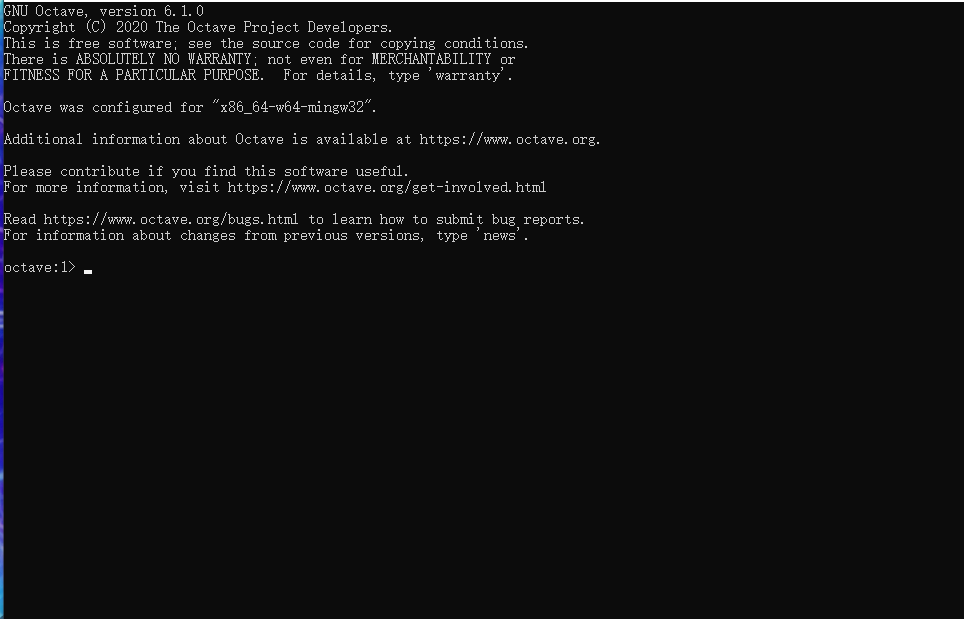基础语法

1. 加减乘除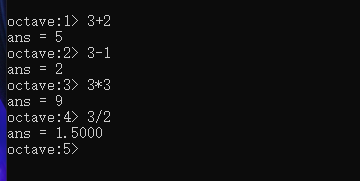2. 逻辑运算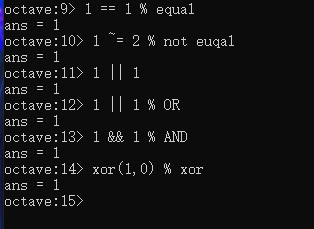% 为注释
3. 变量赋值与显示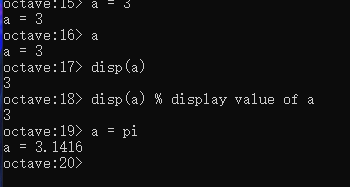4. 矩阵、行向量、列向量、单位矩阵生成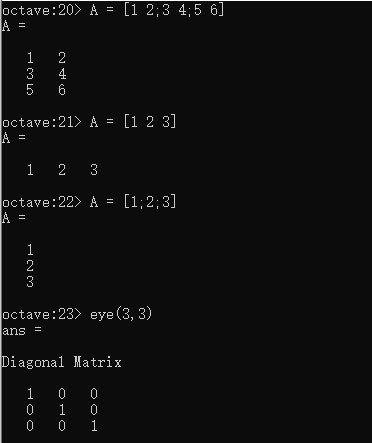5. 随机数、初始化矩阵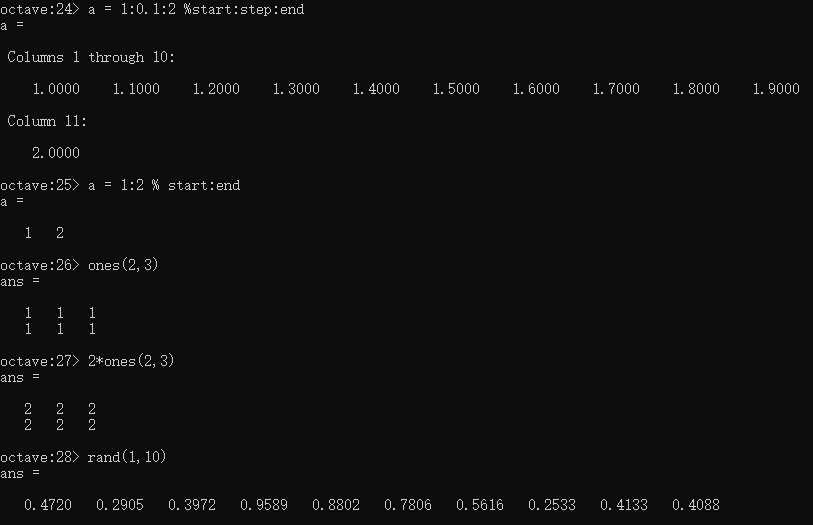6. 简单绘图展示数据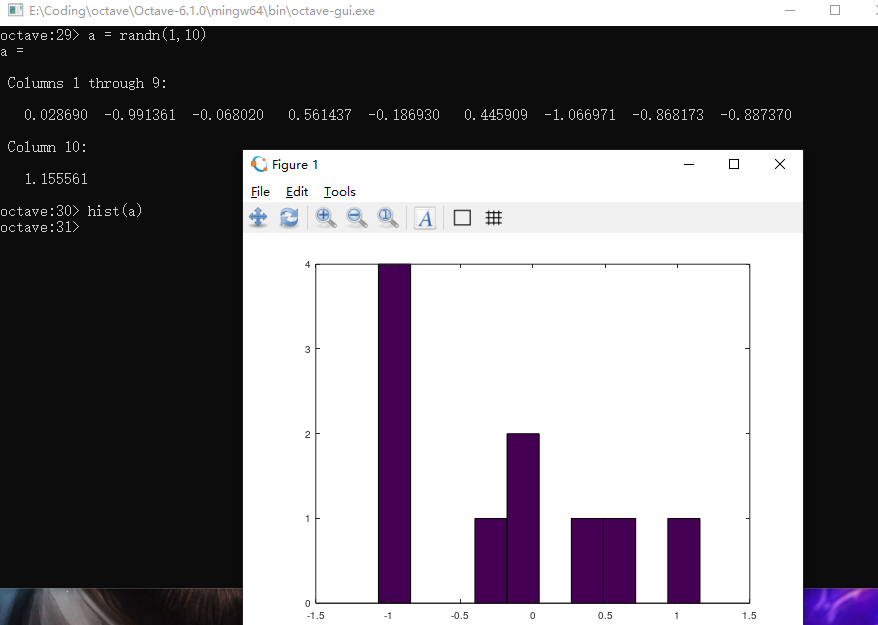展开全文机器学习 编程语言
• 吴恩达《机器学习》课程制定工具Octave的入门教程 吴恩达《机器学习》课程制定工具Octave的入门教程
• Octave是矩阵运算的利器，有人说Octave是Matlab的一个免费版本，个人也是这么认为的。但是Matlab是一个庞然大物，虽然Octave相比之下十分小，但是五脏俱全，可以用来画图，解方程组，这个文档给出了实现这些功能的...
• ## Octave教程（一）

千次阅读 2019-09-29 20:03:56
我将教你一种编程语言：Octave语言。你能够用它来非常迅速地实现这门课中我们已经学过的，或者将要学的机器学习算法。...事实上，在硅谷，我经常看到进行大规模的机器学习项目的人，通常使用的程序语言就是Octave...

我将教你一种编程语言：Octave语言。你能够用它来非常迅速地实现这门课中我们已经学过的，或者将要学的机器学习算法。

过去我一直尝试用不同的编程语言来教授机器学习，包括C++JavaPythonNumpyOctave。我发现当使用像Octave这样的高级语言时，学生能够更快更好地学习并掌握这些算法。事实上，在硅谷，我经常看到进行大规模的机器学习项目的人，通常使用的程序语言就是Octave。(编者注：这是当时的情况，现在主要是用Python)

Octave是一种很好的原始语言(prototyping language)，使用Octave你能快速地实现你的算法，剩下的事情，你只需要进行大规模的资源配置，你只用再花时间用C++Java这些语言把算法重新实现就行了。开发项目的时间是很宝贵的，机器学习的时间也是很宝贵的。所以，如果你能让你的学习算法在Octave上快速的实现，基本的想法实现以后，再用C++或者Java去改写，这样你就能节省出大量的时间。

据我所见，人们使用最多的用于机器学习的原始语言是OctaveMATLABPythonNumPy 和R

Octave很好，因为它是开源的。当然MATLAB也很好，但它不是每个人都买得起的。(貌似国内学生喜欢用收费的matlabmatlab功能要比Octave强大的多，网上有各种D版可以下载)。这次机器学习课的作业也是用matlab的。如果你能够使用matlab，你也可以在这门课里面使用。

如果你会PythonNumPy或者R语言，我也见过有人用 R的，据我所知，这些人不得不中途放弃了，因为这些语言在开发上比较慢，而且，因为这些语言如：PythonNumPy的语法相较于Octave来说，还是更麻烦一点。正因为这样，所以我强烈建议不要用NumPy或者R来完整这门课的作业，我建议在这门课中用Octave来写程序。

本视频将快速地介绍一系列的命令，目标是迅速地展示，通过这一系列Octave的命令，让你知道Octave能用来做什么。

启动Octave

现在打开Octave，这是Octave命令行。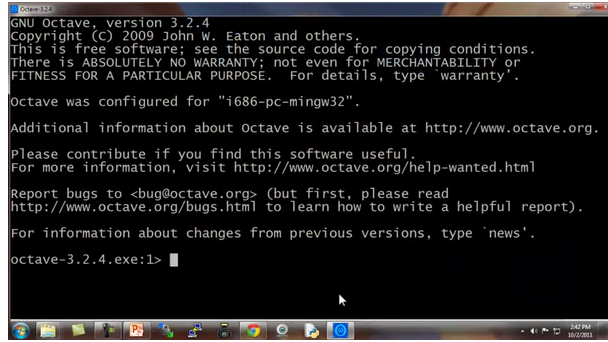现在让我示范最基本的Octave代码：

输入5 + 6，然后得到11。

输入3 – 2、5×8、1/2、2^6等等，得到相应答案。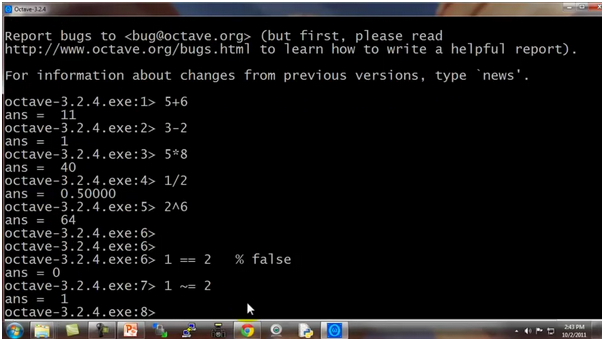这些都是基本的数学运算。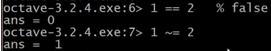你也可以做逻辑运算，例如 1==2，计算结果为 false ()，这里的百分号命令表示注释，1==2 计算结果为假，这里用0表示。

请注意，不等于符号的写法是这个波浪线加上等于符号 ( ~= )，而不是等于感叹号加等号( != )，这是和其他一些编程语言中不太一样的地方。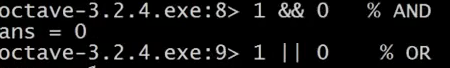让我们看看逻辑运算 1 && 0，使用双&符号表示逻辑与，1 && 0判断为假，1和0的或运算 1 || 0，其计算结果为真。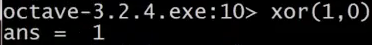还有异或运算 如XOR ( 1, 0 )，其返回值为1

从左向右写着 Octave 324.x版本，是默认的Octave提示，它显示了当前Octave的版本，以及相关的其它信息。

如果你不想看到那个提示，这里有一个隐藏的命令：

输入命令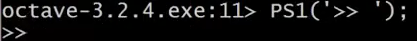现在命令提示已经变得简化了。

接下来，我们将谈到Octave的变量。

现在写一个变量，对变量$A$赋值为3，并按下回车键，显示变量$A$等于3。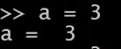如果你想分配一个变量，但不希望在屏幕上显示结果，你可以在命令后加一个分号，可以抑制打印输出，敲入回车后，不打印任何东西。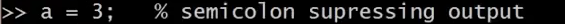其中这句命令不打印任何东西。

现在举一个字符串的例子：变量$b$等于"hi"。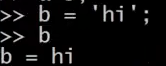$c$等于3大于等于1，所以，现在$c$变量的值是真。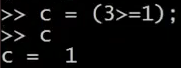如果你想打印出变量，或显示一个变量，你可以像下面这么做：

设置$a$等于圆周率$π$，如果我要打印该值，那么只需键入a像这样 就打印出来了。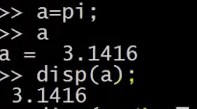对于更复杂的屏幕输出，也可以用DISP命令显示：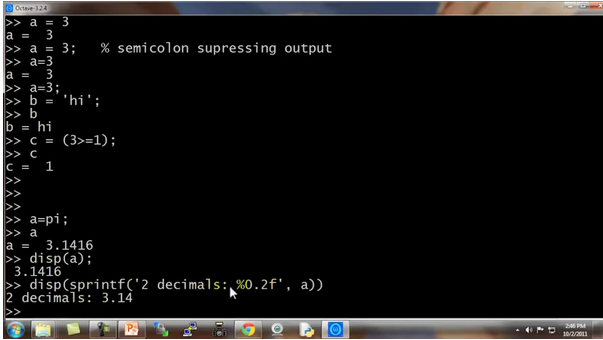这是一种，旧风格的C语言语法，对于之前就学过C语言的同学来说，你可以使用这种基本的语法来将结果打印到屏幕。

例如 ^{T}命令的六个小数：0.6%f ,a，这应该打印$π$的6位小数形式。

也有一些控制输出长短格式的快捷命令：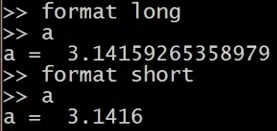展开全文• 老师推荐人工智能最简单入手的是octave，用它写出来之后再转用python、c++会更方便。 简介 GNU Octave is a high-level language primarily intended for numerical computations. It is typically used for such ...

### 文章目录

老师推荐人工智能最简单入手的是octave，用它写出来之后再转用python、c++会更方便。

# 简介

GNU Octave is a high-level language primarily intended for numerical computations. It is
typically used for such problems as solving linear and nonlinear equations, numerical linear
algebra, statistical analysis, and for performing other numerical experiments. It may also
be used as a batch-oriented language for automated data processing.

GNU Octave是一种高级语言，主要用于数值计算。它通常用于求解线性和非线性方程、数值线性代数、统计分析等问题，以及进行其他数值实验。它也可以用作自动化数据处理的面向批处理的语言。

这只是做个简单入门，更多的功能可以看一下官方文档：octave.pdf

# 基本

1. 注释 %

• 类似于C++的//和python的#
•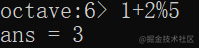2. ans 用于存储输出结果

3. 如果你不喜欢输入行前缀，可以自定义修改PS1('你想改成的内容');

•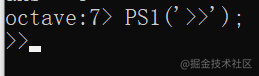这个不是永久的，你电脑重启之后他又恢复默认了。
4. help 帮助
如果你忘了某一个语句的用法了，可以用这个查询。

比如输入help eye就会显示eye的用法。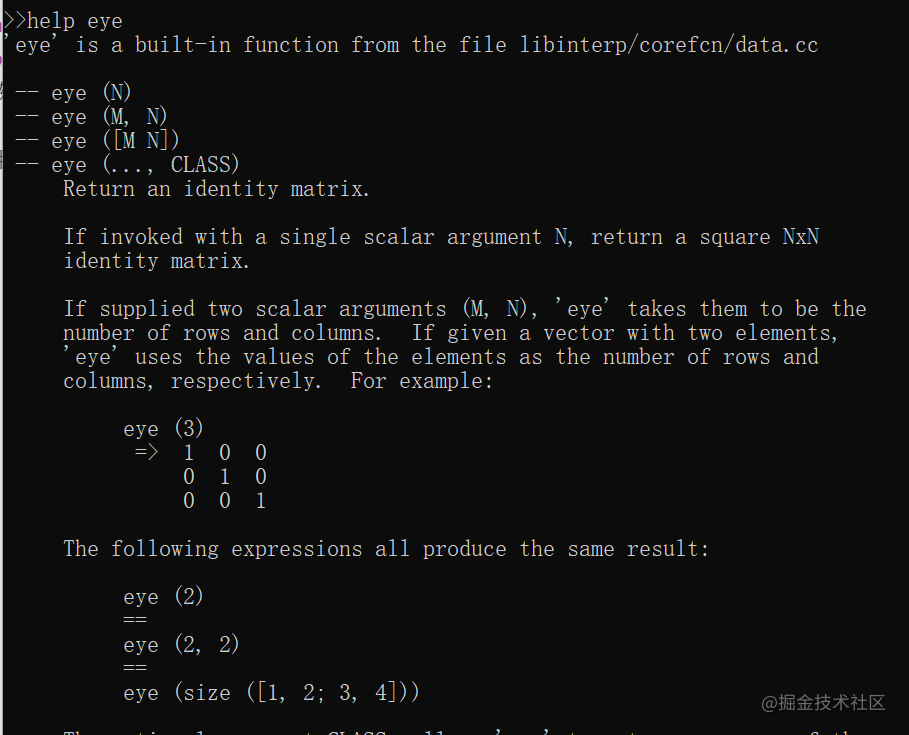5.多个语句同时执行用逗号分开即可。
c octave:226> a=4,b=5,c=6 a = 4 b = 5 c = 6
6. quitexit可以关闭Octave（如果你不想点右上角的×的话）
7. addpath('路径')添加路径

# 符运算

## 算术运算符

加减乘除乘方

octave:1> 1+5
ans = 6
octave:2> 1*5
ans = 5
octave:3> 1/5
ans = 0.2000
octave:4> 1-5
ans = -4
octave:5> 5^2
ans = 25


## 逻辑运算符

• 等于 ==
• 不等于~=
• ||
• &&
• 异或 xor(a,b)
• 大于等于>=
• 大于 >
ans = 0
>>1==1
ans = 1
>>1 ~=2
ans = 1
>>1&&2
ans = 1
>>1&&0
ans = 0
>>1||0
ans = 1
>>xor(1,1)
ans = 0
>>xor(1,2)
ans = 0
>>xor(0,1)
ans = 1
>>1>=2
ans = 0
>>1<2
ans = 1


## 赋值运算符

• =赋值运算符
• 语句后边加分号 不直接打印赋值语句
• 语句后边不加分号 直接打印赋值语句
>>a=1   %a=1后边没分号，所以输入回车之后下一行会直接打印a=1
a = 1
>>a     %再输入a回车看看是否赋值成功
a = 1
>>b=2;  %b=2后边加上分号了，所以下一行不会直接打印b的赋值
>>b     %输入b回车，查看给b的赋值
b = 2

>>c=pi
c = 3.1416


## 格式化输出

• disp(x)：打印x的值
• disp(sprintf('%0.2f',c))：格式化输出保留两位小数，这个和C语言一样
• format long/short：对整数无影响，对小数输出格式有影响
>>disp(a)
1
>>disp(c)
3.1416
>>disp(sprintf('%0.2f',c))
3.14

>>format long
>>a
a = 1
>>c
c = 3.141592653589793
>>format short
>>a
a = 1
>>c
c = 3.1416


## 向量和矩阵

声明矩阵官方原话写的是：

Vectors and matrices are the basic building blocks for numerical analysis. To create a new matrix and store it in a variable so that you can refer to it later, type the command

octave:1> A = [ 1, 1, 2; 3, 5, 8; 13, 21, 34 ]

Octave will respond by printing the matrix in neatly aligned columns.

>>martix = [1,2,3;4,5,6;7,8,9]
martix =

1   2   3
4   5   6
7   8   9


当然还有很多其他写法，虽然不规范也能声明矩阵，这里就不列举了。

声明行向量，各个元素之间不用加符号，或者加逗号。

martix =

1   2
2   3
3   4

>>vector=[1 2 3]
vector =

1   2   3

>>vector = [4;5;6]
vector =

4
5
6


其他特殊写法

• 变量 = 起始:终止 ，生成一个向量，并且步长为1

• 变量 = 起始:步长:终止 ，就会生成一个向量

> v = 1:5
v =

1   2   3   4   5

> m = 1:0.5:1
m = 1
octave:3> m = 1:0.5:5
m =

1.0000    1.5000    2.0000    2.5000    3.0000    3.5000    4.0000    4.5000    5.0000

• ones(a,b) 生成a行b列只有1的矩阵

• 除了ones还有zeros，生成的元素都是0，但是没有twos、threes……
•   >ones(2,3)
ans =

1   1   1
1   1   1

• rand(n) 形成n阶方阵，元素都是0-1之间随机数

• rand(a,b) 生成a行b列的0-1之间随机数的矩阵

•   >rand(2,3)
ans =

0.4046   0.4508   0.8021
0.6986   0.8620   0.2631

• max(rand(n),rand(n))生成量个方阵，取其中较大值形成一个新方阵
octave:124> c = max(rand(4),rand(4))
c =

0.8010   0.9926   0.7241   0.9053
0.9007   0.3026   0.9856   0.5710
0.7679   0.2630   0.5200   0.9615
0.7333   0.5113   0.6957   0.5683

• randn(a,b) 也是生成a行b列随机数矩阵，但是符合高斯分布（正态分布）。返回一个具有正态分布随机元素的矩阵，这些随机元素的平均值为零，方差为1。

• 正态分布：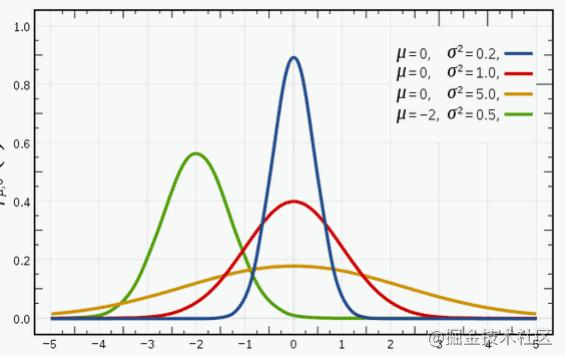• randi()

• randi(max,m,n)：生成1~max的m行n列矩阵
• randi([min,max],m,n)：生成min~max的m行n列矩阵
•   >randi(5,3,3)
ans =

1   5   5
5   2   1
5   1   1

•   >randi([5,10],5,5)
ans =
6    8    6    8    5
10    9   10    8    7
5    7    7    9   10
10    8   10    8    8
10    9    7    6    7


• eye(n) 生成n维单位矩阵

>eye(5)
ans =

Diagonal Matrix

1   0   0   0   0
0   1   0   0   0
0   0   1   0   0
0   0   0   1   0
0   0   0   0   1

• matrixB = matrixA(x:y,v:w):将matrixA的x~y行v~w列形成一个新矩阵

• :表示所有元素
• a:b表示a到b的元素
• [a b c]表示abc三行或列
• 以上三种表示方法在矩阵别的方法中也能使用，比如赋值、打印
• 矩阵拼接

• 在矩阵右侧添加一列
octave:30> A
A =

4    2    7    5
1   10    7   10
5   10    9    9

octave:31> A = [A,[2;4;5]]
A =

4    2    7    5    2
1   10    7   10    4
5   10    9    9    5

• matrixC=[matrixA matrixB] 横向拼接
[matrixA, matrixB]也一样，加不加逗号都行
octave:33> A = randi(5,2,3)
A =

4   1   3
2   3   5

octave:34> B = randi(5,2,3)
B =

5   4   5
1   1   3

octave:35> c = [A B]
c =

4   1   3   5   4   5
2   3   5   1   1   3

• matrixC=[matrixA; matrixB]纵向拼接
octave:33> A = randi(5,2,3)
A =

4   1   3
2   3   5

octave:34> B = randi(5,2,3)
B =

5   4   5
1   1   3

octave:36> d=[A;B]
d =

4   1   3
2   3   5
5   4   5
1   1   3

• matrix(:) 将矩阵中的所有元素放入一个列向量

octave:30> A
A =

4    2    7    5
1   10    7   10
5   10    9    9

octave:31> A(:)
ans =

4
1
5
2
10
10
7
7
9
5
10
9
2
4
5


## 其他

• hist(变量)

可以画出变量的图像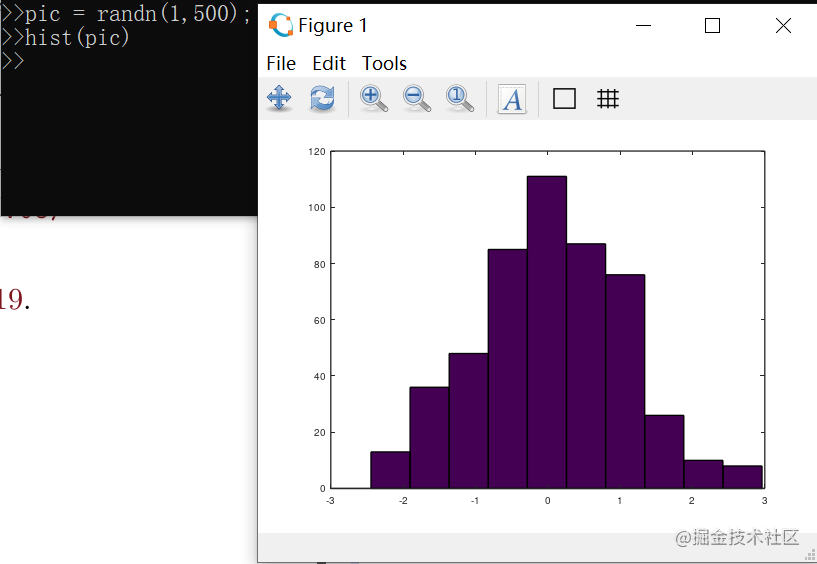如果你觉得这样太少了。那可以使用hist(变量，条纹数)，下图就是画出50条纹后的图像。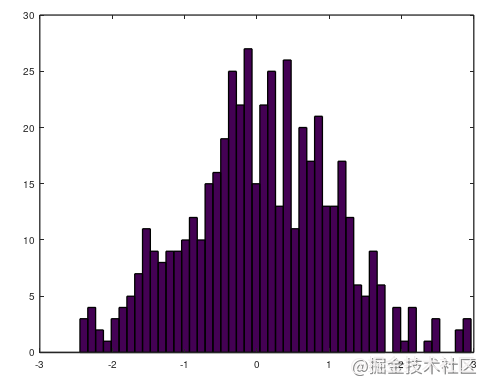• 检测大小

• size()
• length()

下图可以看出，length显示矩阵的长度。size显示矩阵有几行几列。size(矩阵名称，1/2)其中1代表行参数，2代表列参数。

v =

9    9    5    8    8
5   10    7    6    8
6    7    7    7    6
7   10    8    7    9
7   10    7    5    6

>length (v)
ans = 5
>size(v)
ans =

5   5
>size(v,1)
ans = 5

• 索引：

• matrix(i,j) 显示第i行第j列的元素
octave:26> A = randi(10,3,4) %生成一个1~10之间随机数的 3×4 的矩阵
A =
4    2    7    5
1   10    7   10
5   10    9    9
octave:27> A(2,3)            %打印这个矩阵2行3列的元素
ans = 7

• matrix(:,[a,b,c...])
• :：代表全部
• []：方括号中有哪几个就选中哪几个
•   octave:26> A = randi(10,3,4)
A =

4    2    7    5
1   10    7   10
5   10    9    9

octave:27> A(:,[1,2,4])   %显示A所有行的1,2,4列
ans =

4    2    5
1   10   10
5   10    9

• 显示现有变量

• who：显示目前所有变量
• whos：显示目前所有变量和详细信息
•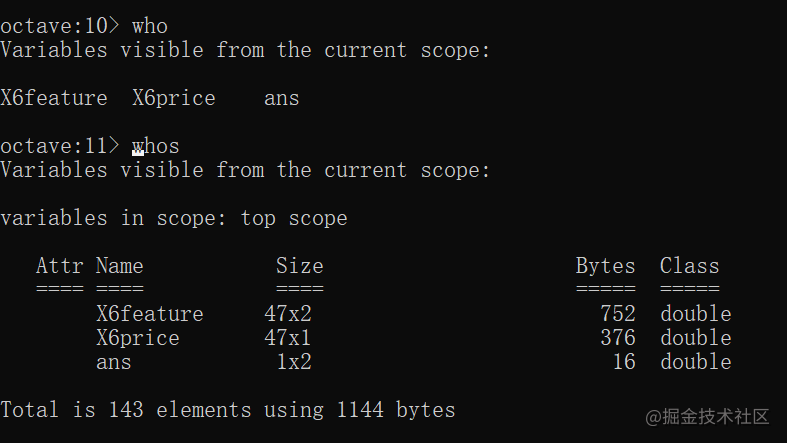• 删除某个变量 clear

• clear 变量 删除三个变量
• clear 删除所有变量
octave:12> a = 15   % 给a赋值15
a = 15
octave:13> a        % 打印a
a = 15              % 显示a的值
octave:14> clear a
octave:15> a        % 再次打印a
error: 'a' undefined near line 1, column 1    %报错


# 移动数据

用过其他命令行的应该能适应，octave也同样支持某些命令。

• pwd：列出当前目录
• cd xxx文件夹：进入xxx文件夹
• ls：列出当前目录信息
• 加载文件：
• load 文件名
• load('文件名')
• save 文件名 变量; ：将变量保存到文件中，比如save data.txt num，把num变量保存到data.txt中（如果操作目录中没有这个文件会创建一个新文件，如果目录中有这个文件会重写）

下边给出两个文件的数据，featureX和priceY，自己搞两个文件存起来。
featureX:

2104  3
1600  3
2400  3
1416  2
3000  4
1985  4
1534  3
1427  3
1380  3
1494  3
1940  4
2000  3
1890  3
4478  5
1268  3
2300  4
1320  2
1236  3
2609  4
3031  4
1767  3
1888  2
1604  3
1962  4
3890  3
1100  3
1458  3
2526  3
2200  3
2637  3
1839  2
1000  1
2040  4
3137  3
1811  4
1437  3
1239  3
2132  4
4215  4
2162  4
1664  2
2238  3
2567  4
1200  3
852  2
1852  4
1203  3


priceY:

399900
329900
369000
232000
539900
299900
314900
198999
212000
242500
239999
347000
329999
699900
259900
449900
299900
199900
499998
599000
252900
255000
242900
259900
573900
249900
464500
469000
475000
299900
349900
169900
314900
579900
285900
249900
229900
345000
549000
287000
368500
329900
314000
299000
179900
299900
239500


首先进入对应的操作目录。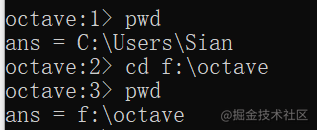用who查看当前有的变量。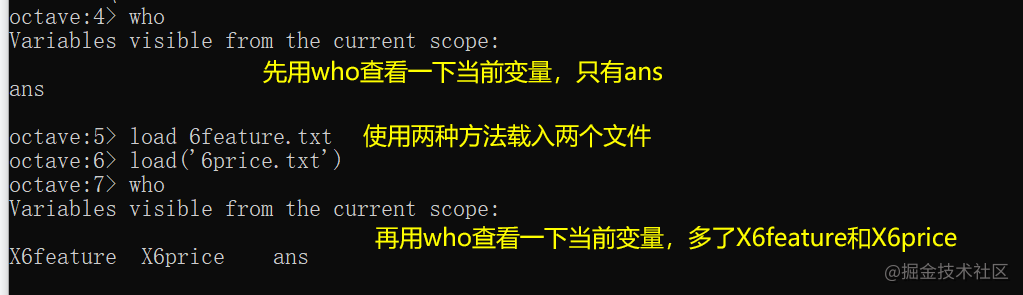打印依稀下X6price确实已经成功读入文件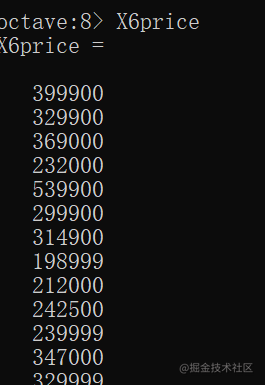用size查看一下，导入的数据是存在矩阵中的。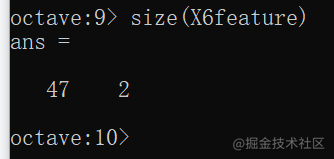把矩阵X6feature的1~5行，第2列 赋值给矩阵x，并将矩阵x保存到data.txt中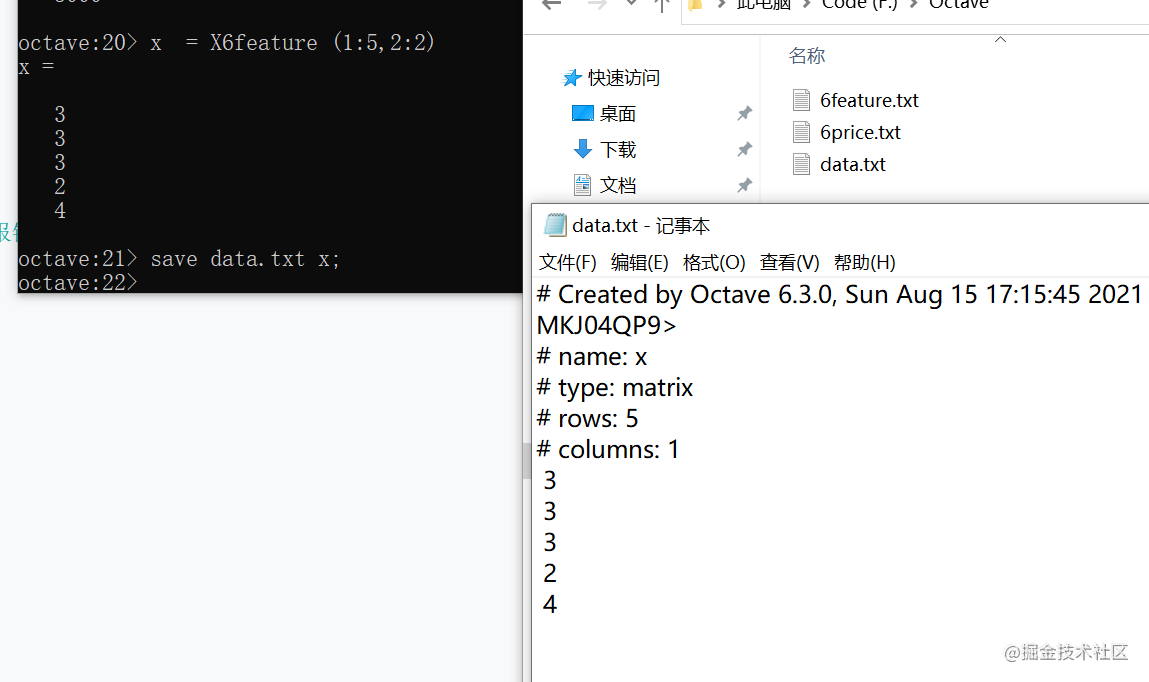# 计算

除了最开始提到的算术运算符，还有以下运算。

注意一个特殊的.*，矩阵同位相乘，即矩阵相同位置上的元素相乘。

octave:37> A
A =

4   1   3
2   3   5

octave:38> B
B =

5   4   5
1   1   3

octave:39> A * B    %AB无法相乘
error: operator *: nonconformant arguments (op1 is 2x3, op2 is 2x3)
octave:40> A .* B   %AB可以点乘
ans =

20    4   15
2    3   15


.^给矩阵每个元素做乘方

octave:43> A
A =

4   1   3
2   3   5

octave:44> A .^2
ans =

16    1    9
4    9   25

octave:45>


矩阵/数即可让矩阵的每个元素都除以该数字，但是如果你想让一个数除以矩阵的每个元素，结果仍旧是矩阵，那就需要./

octave:38> B
B =

5   4   5
1   1   3
octave:50> 2 ./B
ans =

0.4000   0.5000   0.4000
2.0000   2.0000   0.6667


如果想让矩阵每个元素都加或减去一个数，直接用+-即可。

octave:71> C
C =

2  -1  -4   1
-5  -2  -1  -1
1  -1  -5  -4

octave:72> C+1
ans =

3   0  -3   2
-4  -1   0   0
2   0  -4  -3

octave:73> C-2
ans =

0  -3  -6  -1
-7  -4  -3  -3
-1  -3  -7  -6

octave:74> 1+C
ans =

3   0  -3   2
-4  -1   0   0
2   0  -4  -3


log(n)这里的log底数是e，即 log ⁡ e n \log_en ln ⁡ n \ln n

octave:57> log(2)
ans = 0.6931
octave:58> log(e)
ans = 1
octave:59> A
A =

4   1   3
2   3   5

octave:60> log(A)
ans =

1.3863        0   1.0986
0.6931   1.0986   1.6094


exp(n)相当于执行 e n e^n

octave:61> exp(1)
ans = 2.7183
octave:62> exp(2)
ans = 7.3891
octave:63> A
A =

4   1   3
2   3   5

octave:64> exp(A)
ans =

54.5982     2.7183    20.0855
7.3891    20.0855   148.4132


abs()求绝对值

octave:66> C = randi([-5,2],3,4)    %随机生成一个3×4的矩阵，取值在-5\~2之间
C =

2  -1  -4   1
-5  -2  -1  -1
1  -1  -5  -4

octave:67> abs(-1)
ans = 1
octave:68> abs(C)
ans =

2   1   4   1
5   2   1   1
1   1   5   4


matrix'转置，矩阵名称后加英文单引号

octave:75> C
C =

2  -1  -4   1
-5  -2  -1  -1
1  -1  -5  -4

octave:76> C'
ans =

2  -5   1
-1  -2  -1
-4  -1  -5
1  -1  -4


flipud使矩阵垂直翻转。就是左边变右边，右边变左边。

result1 =

17    0    0    0    0
0    5    0    0    0
0    0   13    0    0
0    0    0   21    0
0    0    0    0    9

octave:140> flipud(result1)
ans =

0    0    0    0    9
0    0    0   21    0
0    0   13    0    0
0    5    0    0    0
17    0    0    0    0


矩阵<数值大于啊等于啊都同理。会返回一个同纬度的矩阵，每个元素都是对应位置元素进行比较返回的布尔值

a =

1   5   3   4

octave:85> a<2
ans =

1  0  0  0


magic(n)生成n阶幻方，所谓幻方就是行、列、对角线加起来都是相同的值

octave:95> magic(4)
ans =

16    2    3   13
5   11   10    8
9    7    6   12
4   14   15    1

octave:96> magic(3)
ans =

8   1   6
3   5   7
4   9   2


find(公式)

• 找到向量中符合的数据并返回其索引
a =

1   5   3   4

octave:88> find(a<3)
ans = 1
octave:89> find(a>2)
ans =

2   3   4

• 找到矩阵中符合的数据
C =

9    7    3    5
4    2    5   10
4    1    9   10

octave:114> [r,c]=find(C==1)
r = 3
c = 2
octave:115> [r,c]=find(C>7)
r =

1
3
2
3

c =

1
3
4
4


sum()求和prod()求积

c =

1
3
4
4

octave:117> sum(c)
ans = 12
octave:118> prod(c)
ans = 48


floor()向下取整ceil()向上取整

a =

0.5000
1.5000
2.0000
2.0000

octave:120> floor(a)
ans =

0
1
2
2

octave:121> ceil(a)
ans =

1
2
2
2



max(矩阵)取最大值
如果是矩阵，那就显示每一列的最大值；如果是向量，那就只显示一个最大值

• v=max(matrix)：将矩阵的最大值赋给v，不是硬性要求，v可以换别的名称
• [v,i]=max(matrix)：将矩阵最大值及其位置赋值给v和i
• max(max(matrix))：取得整个矩阵最大值
• max(matrix(:))：取得整个矩阵最大值，这个是将矩阵转化成响亮之后再求最大值
c =

2   1   4   1
5   2   1   1
1   1   5   4

octave:78> val = max(c)
val =

5   2   5   4

octave:79> [val,ind]=max(c)
val =

5   2   5   4

ind =

2   2   3   3


max(矩阵,[],1/2) 取得矩阵每一行或者列的最大值形成一个向量

• 1 取每一列
• 2 取每一行
c =

8   9   7   9
9   3   9   5
7   2   5   9
7   5   6   5

octave:129> max(c,[],1)
ans =

9   9   9   9

octave:130> max(c,[],2)
ans =

9
9
9
7


小练习：
生成一个幻方，然后测试其每行，每列，对角线之和都是同一个数

octave:131>  m = magic(5)     %生成一个5×5的幻方
m =

17   24    1    8   15
23    5    7   14   16
4    6   13   20   22
10   12   19   21    3
11   18   25    2    9

octave:132> sum(m,1)          %每列相加都是65
ans =

65   65   65   65   65

octave:133> sum(m,2)          %每行相加都是65
ans =

65
65
65
65
65

octave:135> e=eye(5)          %生成一个单位矩阵
e =

Diagonal Matrix

1   0   0   0   0
0   1   0   0   0
0   0   1   0   0
0   0   0   1   0
0   0   0   0   1

octave:136> result1 = e .* m   %单位矩阵同位相乘原矩阵，新矩阵只剩主对角线元素
result1 =

17    0    0    0    0
0    5    0    0    0
0    0   13    0    0
0    0    0   21    0
0    0    0    0    9
octave:139> sum(result1(:))   %主对角线相加为65
ans = 65

octave:141> result2 = m .* flipud(e)
result2 =

0    0    0    0   15
0    0    0   14    0
0    0   13    0    0
0   12    0    0    0
11    0    0    0    0

octave:142> sum(result2(:))   %副加为65
ans = 65


pinv()求逆矩阵

m =

8   1   6
3   5   7
4   9   2

octave:145> pinv(m)
ans =

0.147222  -0.144444   0.063889
-0.061111   0.022222   0.105556
-0.019444   0.188889  -0.102778


# 绘图

plot(A,B)A是横坐标向量，B纵坐标

octave:173> t=[-1:0.01:1];
octave:174> p1 = sin(2*pi*t);
octave:175> plot(t,p1)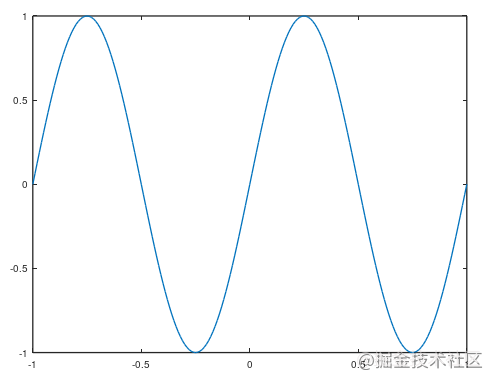octave:176> p2 = cos(2*pi*t);
octave:177> plot(t,p2)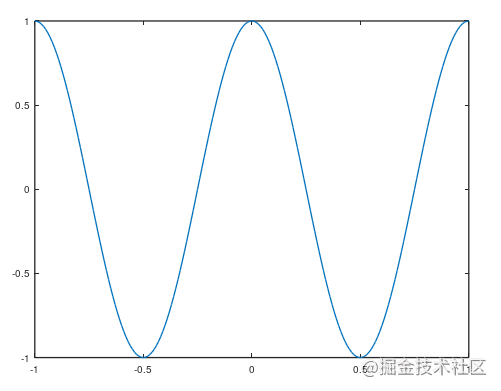画完一张图以后想要画另一张就会重新绘制，用hold on可以保留，在原图基础上继续画，并且会自动给你切换颜色。

octave:177> plot(t,p2)
octave:178> hold on
octave:179> plot(t,p1)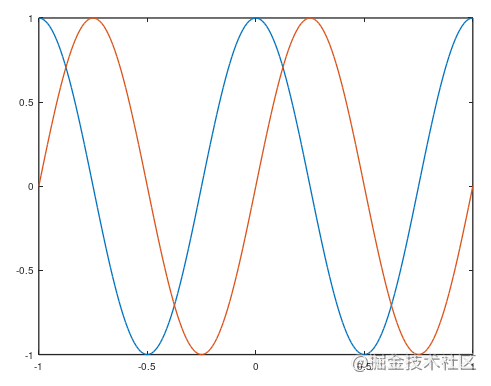给横轴纵轴添加标签。

octave:183> xlabel('time')
octave:184> ylabel('speed')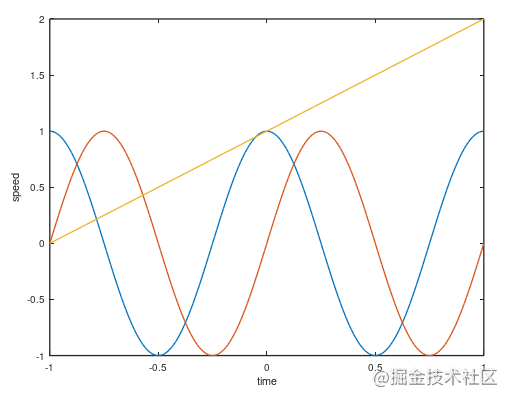添加图例

legend('cos','sin','y')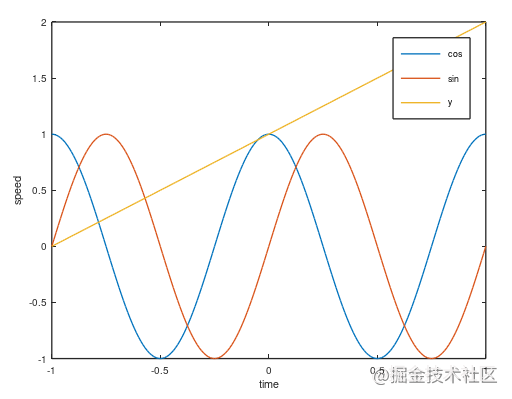添加标题

title('my demo')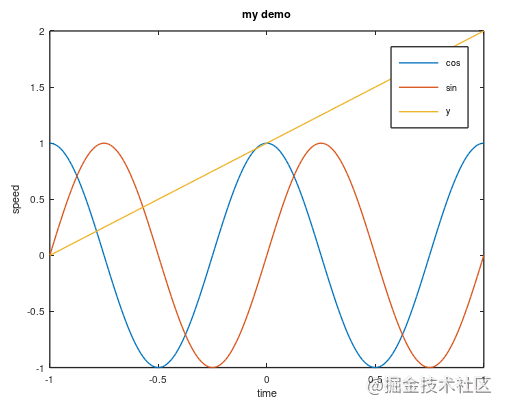保存文件

print -dpng '文件名'


保存之后你的当前目录下边就会多出一个图片。如过你不知道当前目录是啥，输入pwd查看当前目录的路径。

如果你想同时生成多个图片，那在前边加上figure(n);则会按顺序生成图片。

octave:194> figure(1);plot(t,p1)
octave:195> figure(2);plot(t,p2)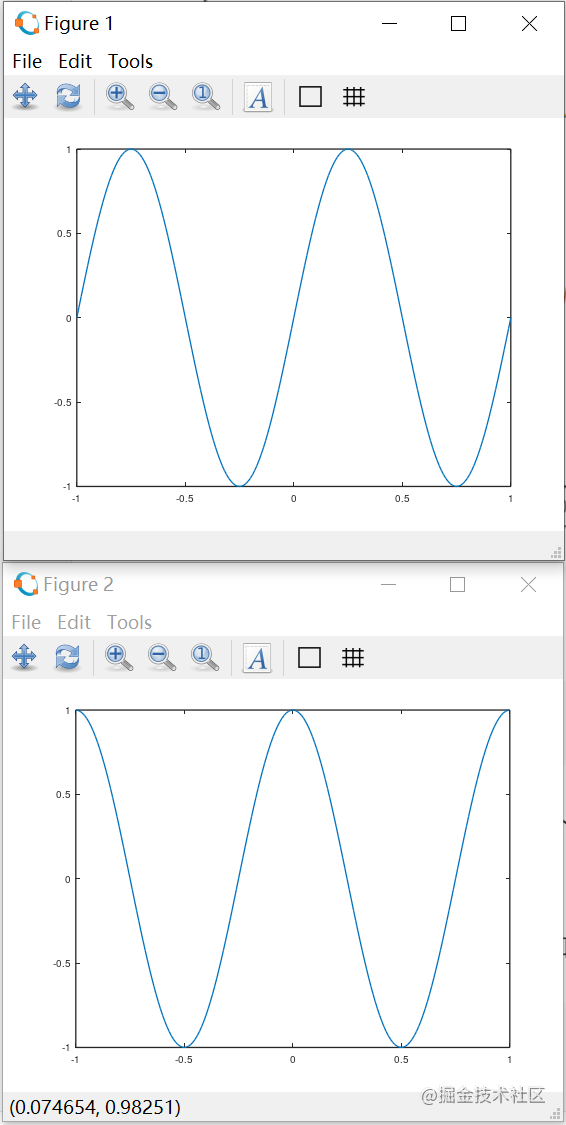合并显示图像subplot(n,m,index)，将图像分成n行m列，取index位置绘图。

octave:197> subplot(2,3,4)
octave:198> plot(t,p1)
octave:199> subplot(2,3,2)
octave:200> plot(t,p2)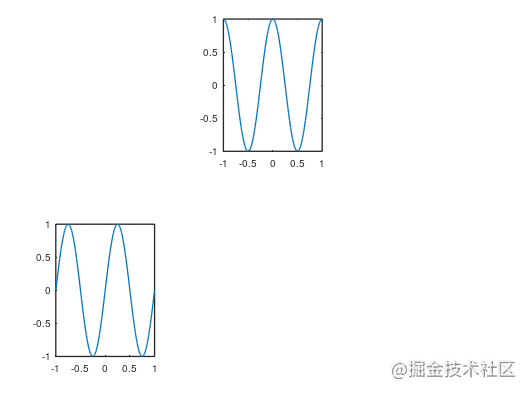改变纵横轴取值范围axis([横轴起始 终止 纵轴起始 终止])

octave:203> subplot(1,2,1)
octave:204> plot(t,p2)
octave:205> subplot(1,2,2)
octave:206> plot(t,p2)
octave:207> axis([0 1 -2 2])


下图两个是同一副图，右边是改变坐标轴范围之后的图像。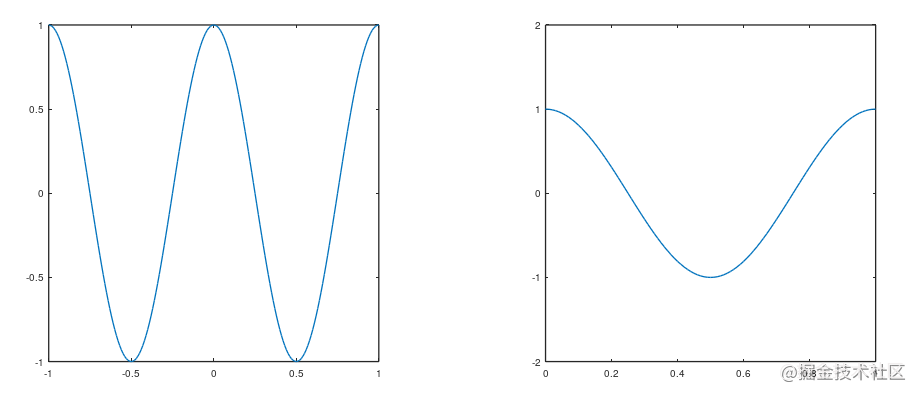clf清空整幅图，close不用点右上角×关闭图像

矩阵可视化
imagesc()

a =

8   1   6
3   5   7
4   9   2

octave:217> imagesc (a)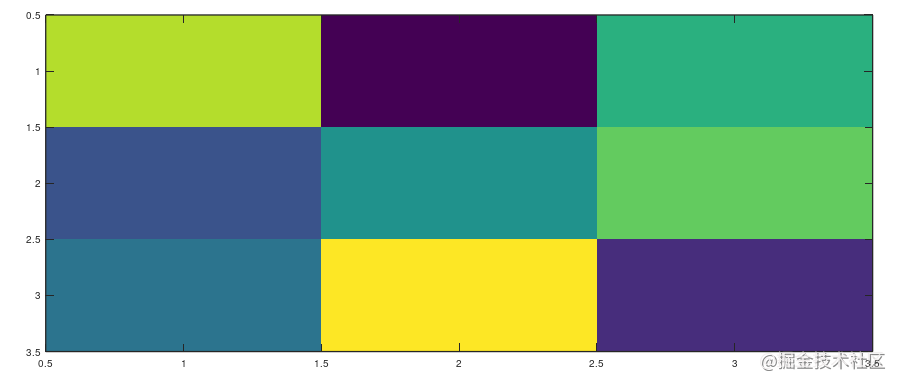说个题外话，作为一个曾经的生物学生，我好像突然get到了我们以前的热图是怎么出来的。

octave:218> load 6feature.txt   % 还记得这组数据吗，就是我上边给的那两组数据的第一组。
octave:219> imagesc (X6feature)


画出来以后图像长这样，恩我真的get到热图怎么画的了。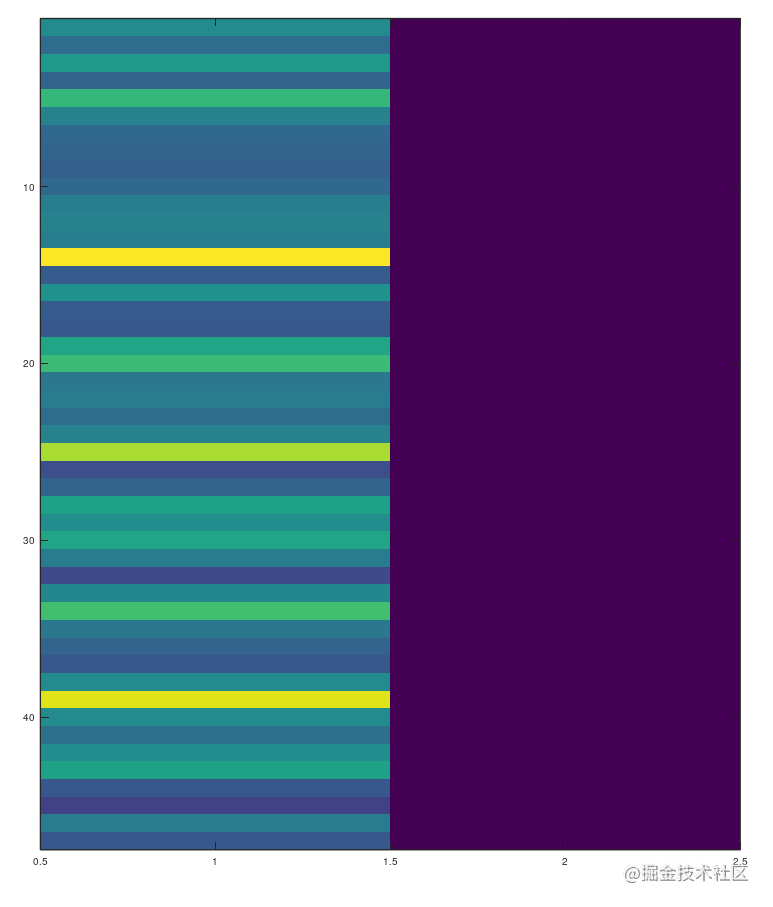现在让我们生成一个热图（假的，不是热图，看起来像而已）

octave:220> r = rand(5,50);
octave:221> imagesc (r)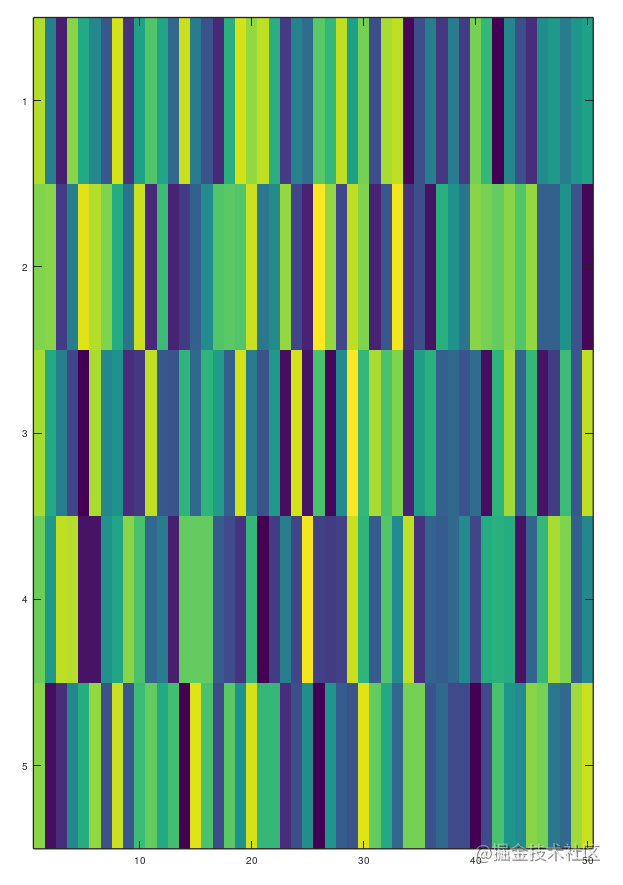colorbar添加比色的图例。

octave:223> colorbar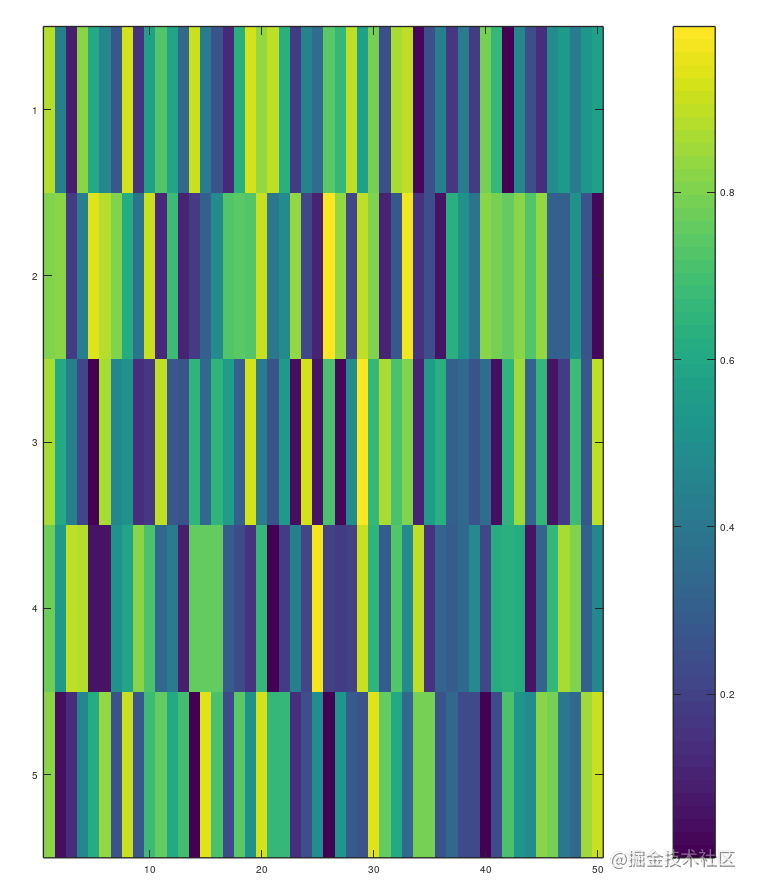colormap gray使其变为灰度图

 colormap gray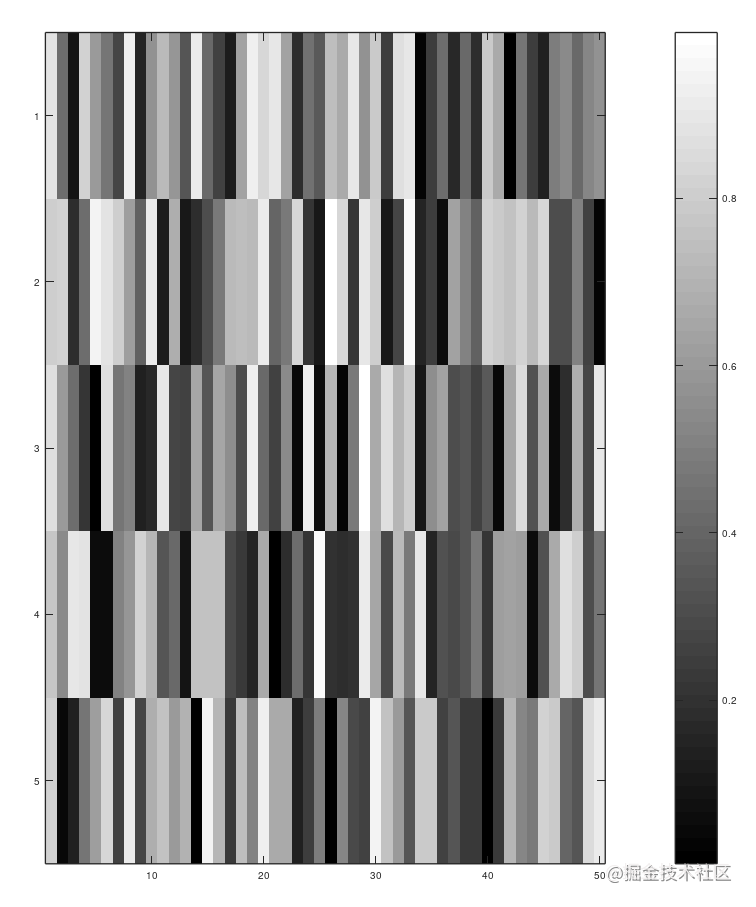# 基础语法

• 循环

for var = expression
body
endfor

while (condition)
body
endwhile

• 其实上边的endwhile，endfor还有下边的endif可以直接写为end
• break、continue也可以用
• 判断

if (condition)
then-body
endif

if (condition)
then-body
elseif (condition)
elseif-body
else
else-body
endif

• 举个栗子

v =

8    4    8    2    5
7    4    6    1    3
10    6    6    4   10
6    6    8    8   10
9    1   10    5    7

> for i = 1:5
>     for j = 1:5
>         if v(i,j)<5
>             continue
>         else
>             disp(v(i,j))
>         endif
>     endfor
> endfor
8
8
5
7
6
10
6
6
10
6
6
8
8
10
9
10
5
7

• 函数

• 普无返回值的函数
function name
body
endfunctio

• 带返回值的函数
function [ret-list] = name (arg-list)
body
endfunction

• 在octave中写函数：

>function say_hi(name)
> str = ['hello' name];
> disp(str)
> endfunction
>say_hi ('Sian')
helloSian

• 让octave使用外部的函数。

• 在当前目录或者path中放你的函数文件
• 文件名就是函数名
• 后缀.m`
• 举个栗子
现在写一个函数show_matrix：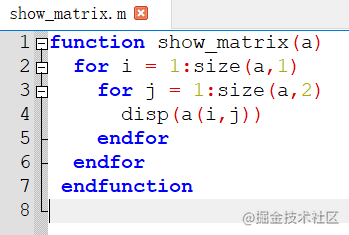存放在path中，并且命名为show_matrix.m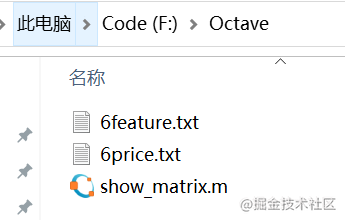回到octave中声明一个矩阵，看看是否能用该函数成功打印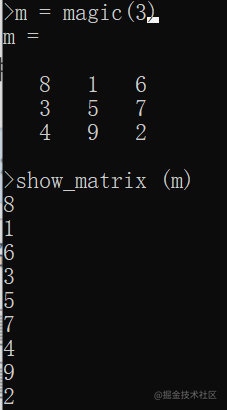• 再整一个带返回值的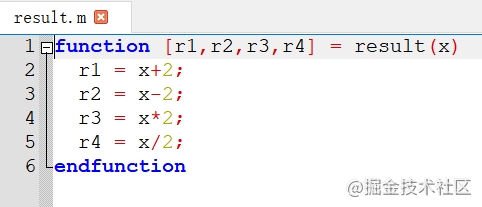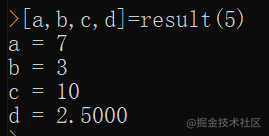展开全文• OCTAVE入门教程 OCTAVE（Operationally Critical Treat，Asset，and Vulnerability Evaluation，可操作的关键威胁、资产和薄弱点评估）是由美国卡耐基·梅隆大学软件工程研究所下属的CERT协调中心开发的用以定义一种...
• octave相较于MATLAB是有开源免费的的优势。 首先，octave命令直接进入环境 加减乘除和其他语言等同理 % 是注释 1 ~= 2 是表示1不等于2，这个和其他语言不同 xor(1,0) 是表示异或 PS1('&gt;&...
• Octave Tutorial Octave教程为什么学习机器学习要学octave等 较高级的原型语言？If you want to build a large scale deployment of a learning algorithm, whatmatlab 机器学习
• https://zhuanlan.zhihu.com/p/28484838python
• 吴恩达老师的课程介绍Octave处理矩阵，几何图形，过滤出独立音频只要用一句代码搞定，所以也过来入门。 下载 下载地址: https://github.com/octave-app/octave-app/releases 下载并安装 更多种安装方式请参考 ...
• 在MAC安装完成Octave后，可以使用octave-gui/octave-cli两个命令打开软件。 一个基本的使用方法是： data = load ("-ascii","export.txt") ﻿hist(data(:,1),50) 注意：如果使用cli模式，会报错弹窗的H没有...
• 希望想学octave的朋友好好学习，该书是一本英文书籍，既可以学到专业知识，又可以提升英语能力
• GNU Octave是一种高级语言，主要设计用来进行数值计算，它...我们甚至可以使用 C 或 C++ 语言编写自己的应用程序，然后与 Octave 进行交互。Octave 最初是在 1992 年作为化学反应堆设计教科书的一个辅助软件而编写的...
• 吴恩达的机器学习课程的作业可以使用Matlab或者octave，这里记录一下octave的基本操作。 Contents Contents 符号 矩阵 矩阵初始化 特殊矩阵初始化 矩阵索引 矩阵变化 重新赋值 添加到新行/列 矩阵向量化 ...机器学习 矩阵 matlab
• 该存储库包含运行Octave Online Server的全部代码，Octave Online Server是为的基础结构。 高层概述 Octave在线服务器包含三个独立的组件： 客户端：在浏览器中运行的代码。 前端服务器：身份验证，客户端会话处理...JavaScript
• 本内容将介绍 Octave 中的矩阵的创建、引用及相关运算。Octave Octave入门 Octave矩阵
• ## Octave绘图教程

千次阅读 2020-07-17 10:03:49
是图例标签 linestyle: '-' 使用实线 '--' 使用虚线 ':' 使用虚线，只不过更密集 '-.' 使用点划线 marker:(描点的符号) '+' 'o' '*' '.' 'x' 's' 一个小正方形 'd' 菱形 '^' 正三角形 'v' 倒三角形 '>' 左...机器学习
• 本内容将介绍 Octave 中的数组的创建和操作。Octave数组 Octave Octave入门
• 本内容将介绍 Octave 中的向量的创建、引用及相关运算。Octave Octave入门
• ## Octave 入门教程

千次阅读 2018-10-18 15:03:08
本内容主要介绍 Octave使用方法。对自己的学习进行总结，同时希望能够对大家有用，谢谢!Octave Octave入门 机器学习
• ## Octave下载与安装教程

千次阅读 多人点赞 2020-12-06 14:05:40
一、Octave介绍 Octave是一种编程语言，旨在解决线性和非线性的数值计算问题。Octave为GNU项目下的开源软件，早期版本为命令行交互方式，4.0.0版本发布基于QT编写的GUI交互界面。Octave语法与Matlab语法非常接近，...
• 本内容将介绍 Octave 的控制语句（if/switch）和循环语句 （for/while/break/continue）的使用方法。Octave Octave入门 机器学习
• 本内容将介绍 Octave 的一些基本操作命令和使用方法。Octave 机器学习 Octave入门
• Octave 安装教程安装路径安装过程安装完成后的提示BLAS库选择随附的Octave软件包questionOctave快速入门 安装路径 https://wiki.octave.org/Octave_for_Microsoft_Windows 简介的文章，以及官方的一些内容。 ...
• GNU Octave是一种高级编程语言，像 MATLAB，它主要是用MATLAB兼容。它也可以用来进行数值计算。Octave 和MATLAB具有以下共同特点：矩阵的基本数据类型它内置支持复数它具有内置数学函数和库它支持用户自定义功能GNU ...
• 1.安装flatpak sudo apt-get install flatpak ...3.使用flatpak安装最新Octave flatpak install flathub org.octave.Octave 两次询问是否要安装，选项均选择y进行安装 这一步一共安装12个包，需要等待较长的时计算机网络 操作系统 NS3...